NEET  >  31 Year NEET Previous Year Questions: Gravitation - 1

# 31 Year NEET Previous Year Questions: Gravitation - 1

Test Description

## 17 Questions MCQ Test Physics Class 11 | 31 Year NEET Previous Year Questions: Gravitation - 1

31 Year NEET Previous Year Questions: Gravitation - 1 for NEET 2023 is part of Physics Class 11 preparation. The 31 Year NEET Previous Year Questions: Gravitation - 1 questions and answers have been prepared according to the NEET exam syllabus.The 31 Year NEET Previous Year Questions: Gravitation - 1 MCQs are made for NEET 2023 Exam. Find important definitions, questions, notes, meanings, examples, exercises, MCQs and online tests for 31 Year NEET Previous Year Questions: Gravitation - 1 below.
Solutions of 31 Year NEET Previous Year Questions: Gravitation - 1 questions in English are available as part of our Physics Class 11 for NEET & 31 Year NEET Previous Year Questions: Gravitation - 1 solutions in Hindi for Physics Class 11 course. Download more important topics, notes, lectures and mock test series for NEET Exam by signing up for free. Attempt 31 Year NEET Previous Year Questions: Gravitation - 1 | 17 questions in 35 minutes | Mock test for NEET preparation | Free important questions MCQ to study Physics Class 11 for NEET Exam | Download free PDF with solutions
 1 Crore+ students have signed up on EduRev. Have you?
31 Year NEET Previous Year Questions: Gravitation - 1 - Question 1

### Two satellites of earth, S1 and S2 are moving in the same orbit. The mass of S1 is four times the mass of S2. Which one of the following statements is true? 

Detailed Solution for 31 Year NEET Previous Year Questions: Gravitation - 1 - Question 1

Since orbital velocity of satellite is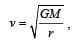it does not depend upon the mass of the satellite.
Therefore, both satellites will move with same speed.

31 Year NEET Previous Year Questions: Gravitation - 1 - Question 2

### A roller coaster is designed such that riders experience “weightlesness” as they go round the top of a hill whose radius of curvature is 20 m.The speed of the car at the top of the hill is between: 

Detailed Solution for 31 Year NEET Previous Year Questions: Gravitation - 1 - Question 2

For the riders to experience weightlessness at the top of the hill, the weight of the radius must be balanced by the centripetal force.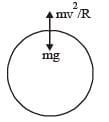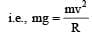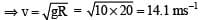Hence, the speed of the car should be between 14 ms–1 and 15 ms–1.

31 Year NEET Previous Year Questions: Gravitation - 1 - Question 3

### The figure shows elliptical or bit of a planet m about the sun S. The shaded area SCD is twice the shaded area SAB. If t1 is the time for the planet to move from C to D and t2 is the time to move from A to B then :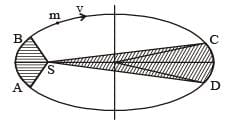Detailed Solution for 31 Year NEET Previous Year Questions: Gravitation - 1 - Question 3

According to Kepler ’s law, the areal velocity of a planet around the sun always remains constant.
SCD : A1–  t1  (areal velocity constant)
SAB : A2 – t2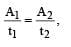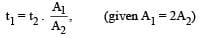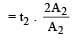∴   t1 = 2t2

31 Year NEET Previous Year Questions: Gravitation - 1 - Question 4

The radii of circular orbits of two satellites A and B of the earth, are 4R and R, respectively. If the speed of satellite A is 3 V, then the speed of satellite B will be: 

Detailed Solution for 31 Year NEET Previous Year Questions: Gravitation - 1 - Question 4

Orbital velocity of a satellite in a circular orbit of radius a is given by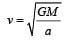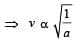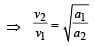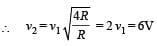31 Year NEET Previous Year Questions: Gravitation - 1 - Question 5

A particle of mass M is situated at the centre of a spherical shell of same mass and radius a. The gravitational potential at a point situated at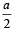distance from the centre, will be: 

Detailed Solution for 31 Year NEET Previous Year Questions: Gravitation - 1 - Question 5

Potential at the given point = Potential at the point due to the shell + Potential due to the particle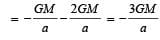31 Year NEET Previous Year Questions: Gravitation - 1 - Question 6

A planet moving along an elliptical orbit is closest to the sun at a distance r1 and farthest away at a distance of r2. If v1 and v2 are the linear velocities at these points respectively, then the ratio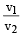is

Detailed Solution for 31 Year NEET Previous Year Questions: Gravitation - 1 - Question 6

Angular momentum is conserved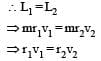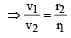31 Year NEET Previous Year Questions: Gravitation - 1 - Question 7

A particle of mass m is thrown upwards from the surface of the earth, with a velocity u. The mass and the radius of the earth are, respectively, M and R. G is gravitational constant and g is acceleration due to gravity on the surface of the earth. The minimum value of u so that the particle does not return back to earth, is [2011M]

Detailed Solution for 31 Year NEET Previous Year Questions: Gravitation - 1 - Question 7

The velocity u should be equal to the escape velocity. That is,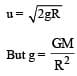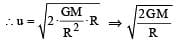31 Year NEET Previous Year Questions: Gravitation - 1 - Question 8

A particle of mass M is situated at the centre of spherical shell of mass M and radius a. The magnitude of the gravitational potential at a point situated at a/2 distance from the centre, will be [2011M]

Detailed Solution for 31 Year NEET Previous Year Questions: Gravitation - 1 - Question 8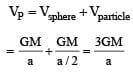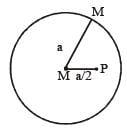31 Year NEET Previous Year Questions: Gravitation - 1 - Question 9

The height at which the weight of a body becomes 1/16th, its weight on the surface of earth (radius R), is : 

Detailed Solution for 31 Year NEET Previous Year Questions: Gravitation - 1 - Question 9

Let at h height, the weight of a body becomes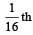of its weight on the surface.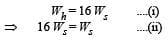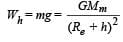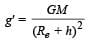Similarly,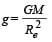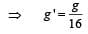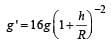h = 3R

31 Year NEET Previous Year Questions: Gravitation - 1 - Question 10

A spherical planet has a mass MP and diameter DP. A particle of mass m falling freely near the surface of this planet will experience an acceleration due to gravity, equal to :  

Detailed Solution for 31 Year NEET Previous Year Questions: Gravitation - 1 - Question 10

Gravitational attraction force on particle B,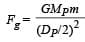Acceleration of particle due to gravity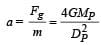31 Year NEET Previous Year Questions: Gravitation - 1 - Question 11

A geostationary satellite is orbiting the earth at a height of 5R above that surface of the earth, R being the radius of the earth. The time period of another satellite in hours at a height of 2R from the surface of the earth is : 

Detailed Solution for 31 Year NEET Previous Year Questions: Gravitation - 1 - Question 11

According to Kelpner’s law of period

T2 ∝ R3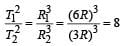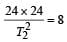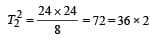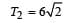31 Year NEET Previous Year Questions: Gravitation - 1 - Question 12

If ve is escape velocity and v0 is orbital velocity of a satellite for orbit close to the earth’s surface, then these are related by : [2012M]

Detailed Solution for 31 Year NEET Previous Year Questions: Gravitation - 1 - Question 12

Escape velocity, ve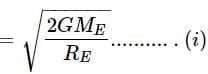Where Mg and RE be the mass and radius of the earth respectively. The orbital velocity of a satallite close to the earth's surface is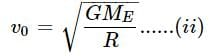From (i) and (ii), we get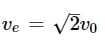31 Year NEET Previous Year Questions: Gravitation - 1 - Question 13

Which one of the following plots represents the variation of gravitational field on a particle with distance r due to a thin spherical shell of radius R ? (r is measured from the centre of the spherical shell) [2012M]

Detailed Solution for 31 Year NEET Previous Year Questions: Gravitation - 1 - Question 13

The Gravitational field due to a thin spherical shell of radius R at distance r.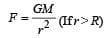For   r = R i.e. on the surface of the shell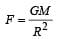For r < R  i.e. inside the shell

F = 0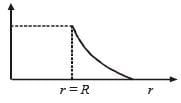31 Year NEET Previous Year Questions: Gravitation - 1 - Question 14

A body of mass ‘m’ is taken from the earth’s surface to the height equal to twice the radius (R) of the earth. The change in potential energy of body will be [NEET 2013]

Detailed Solution for 31 Year NEET Previous Year Questions: Gravitation - 1 - Question 14

Initial P. E.,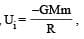Final P.E.,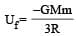[∵ R' = R + 2R = 3R]
∴ Change in potential energy,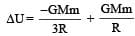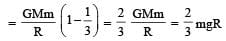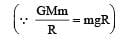ALTERNATE :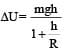By placing the value of h = 2R we get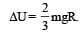31 Year NEET Previous Year Questions: Gravitation - 1 - Question 15

Infinite number of bodies, each of mass 2 kg are situated on x-axis at distances 1m, 2m, 4m, 8m, ..... respectively, from the origin. The resulting gravitational potential due to this system at the origin will be [NEET 2013]

Detailed Solution for 31 Year NEET Previous Year Questions: Gravitation - 1 - Question 15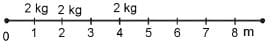Gravitational potential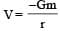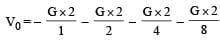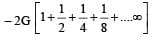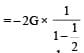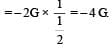31 Year NEET Previous Year Questions: Gravitation - 1 - Question 16

The radius of a planet is twice the radius of earth.Both have almost equal average mass-densities.If VP and VE are escape velocities of the planet and the earth, respectively, then [NEET Kar. 2013]

Detailed Solution for 31 Year NEET Previous Year Questions: Gravitation - 1 - Question 16

Escape velocity,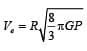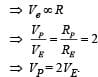31 Year NEET Previous Year Questions: Gravitation - 1 - Question 17

A particle of mass ‘m’ is kept at rest at a height 3R from the surface of earth, where ‘R’ is radius of earth and ‘M’ is mass of earth. The minimum speed with which it should be projected, so that it does not return back, is (g is acceleration due to gravity on the surface of earth) [NEET Kar. 2013]

Detailed Solution for 31 Year NEET Previous Year Questions: Gravitation - 1 - Question 17

As we know, the minimum speed with which a body is projected so that it does not return back is called escape speed.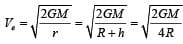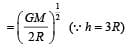## Physics Class 11

127 videos|464 docs|210 tests
 Use Code STAYHOME200 and get INR 200 additional OFF Use Coupon Code
Information about 31 Year NEET Previous Year Questions: Gravitation - 1 Page
In this test you can find the Exam questions for 31 Year NEET Previous Year Questions: Gravitation - 1 solved & explained in the simplest way possible. Besides giving Questions and answers for 31 Year NEET Previous Year Questions: Gravitation - 1, EduRev gives you an ample number of Online tests for practice

## Physics Class 11

127 videos|464 docs|210 tests

### How to Prepare for NEET

Read our guide to prepare for NEET which is created by Toppers & the best Teachers